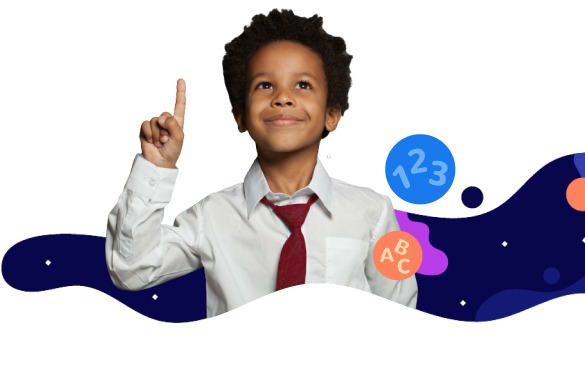## 3rd Graders Multiply by 0 and 1 Educational Resources for Kids

View all 5 resources
•Multiply by 0 and 1
##### Multiply with 0 and 1 Game

Begin the exciting journey of becoming a math wizard by learning how to multiply with 0 and 1.

3 3.OA.5
VIEW DETAILS
•Multiply by 0 and 1
##### Facts of 0 and 1 Game

Take a look at facts of 0 and 1 with this multiplication game.

3 3.OA.5
VIEW DETAILS
• VIEW DETAILS
• VIEW DETAILS

## All 3rd Graders Multiplication Properties Educational Resources for Kids

•##### Multiply with 0 and 1 Game

Begin the exciting journey of becoming a math wizard by learning how to multiply with 0 and 1.

3 3.OA.5
VIEW DETAILS
•##### Facts of 0 and 1 Game

Take a look at facts of 0 and 1 with this multiplication game.

3 3.OA.5
VIEW DETAILS
• VIEW DETAILS
• VIEW DETAILS
•##### Use Facts of 0 and 1 to Find the Missing Number Game

Kids must use the facts of 0 and 1 to find the missing number.

3 3.OA.5
VIEW DETAILS

4413+4567+